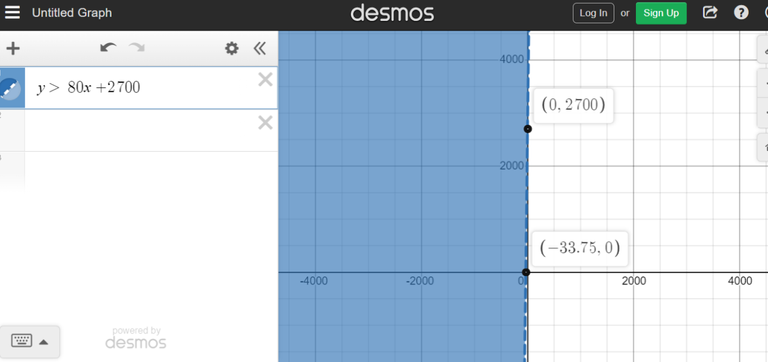# Graphing Linear Inequalities

in StemSocial2 months ago (edited)

Hi there. In this mathematics based post, I cover graphing linear inequalities. It is assumed that the reader is familiar with linear equations and the algebra associated with linear functions.

As usual, I use QuickLatex.com for math text rendering.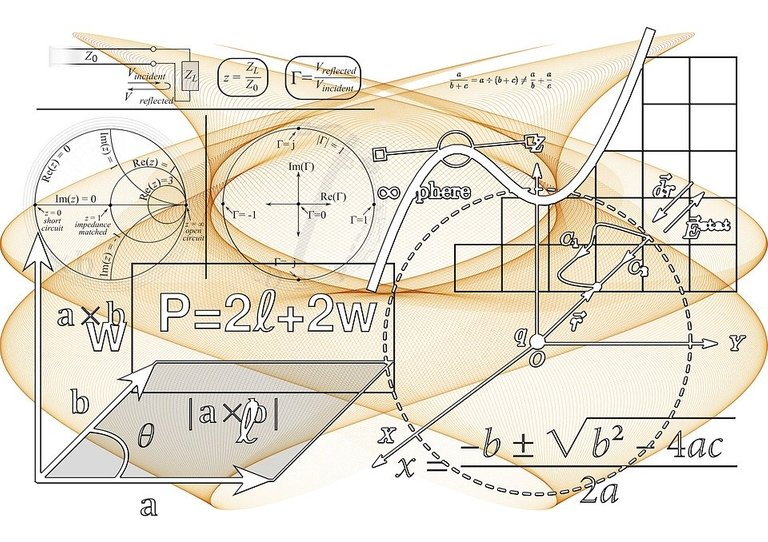Pixabay Image Source

## Topics

• Review Of Linear Equations
• Isolate For y First
• Graph The Inequalities

## Review Of Linear Equations

The equation for a linear function is of the following form: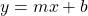The variable `y` is the dependent variable that depends on the value of the independent variable `x`. The value of `b` is the y-intercept or the value of `y` when `x` is 0. To represent the change in `y` for every one unit increase of `x`, we have the slope value `m`.

An example of a linear function is `y = 10x + 20` . I show a screenshot graph below.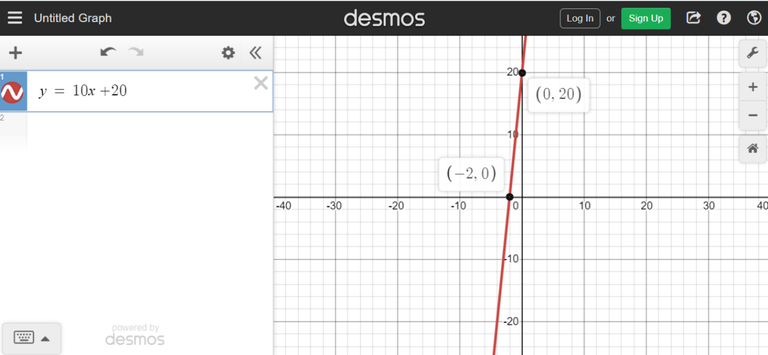## Isolate For y First

Not all equations will have the dependent variable `y` already isolated. This is where algebra comes into play. Isolating for `y` is the key step before graphing the linear inequality.

Example One

Solve for y in `2y - 6 > x`.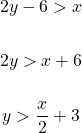The slope for this linear function is one half. Three is the y-intercept here.

Example Two

Isolate for y in `-2x + 5y < 10`.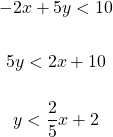With this linear function, the y-intercept is 2 and the slope is two fifths.

Example Three

Isolate for y in `-10y \geq 200`.

When it comes to multiplying or dividing by a negative sign, do make sure to change the direction of the inequality sign.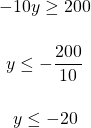## Graph The Inequalities

Once y is isolated, the graphing can commence. Here are some examples.

Example One

In example one from the previous section we obtained `y > x/2 + 3`.

On graphing paper, graph the line `y > x/2 + 3` first. The line should be dashed instead of a solid line. This is because we have a strict inequality. If it was greater or equal to with this symbol ≥ then the line can be solid. As `y` is greater than the line, the shaded region is (vertically) above the line. In the Desmos screenshot below, the shaded region above the dashed line is red.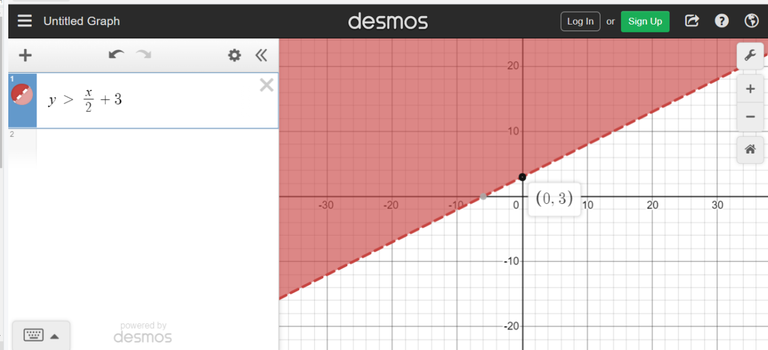Example Two

What would the graph look like for `-2x + 5y < 10` back in example two from the previous section?

Isolating for `y` yielded `y < 2x/5 + 2`.

The line `y < 2x/5 + 2` is graphed first. As the inequality sign is less than (<), the line is dashed. In addition, the region below the line is shaded.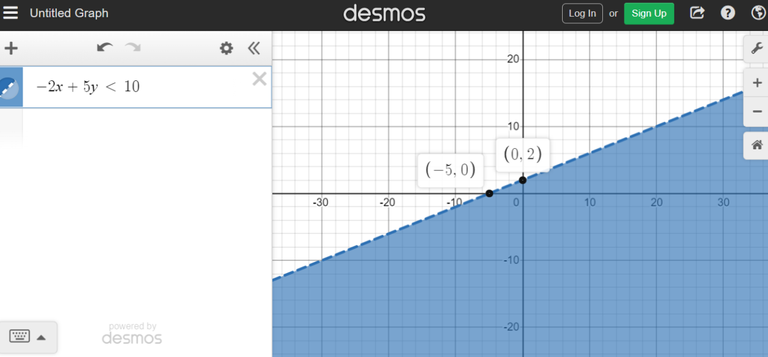Example Three

Graph the inequality `-2y + 9x ≥ -2`.

With this do be careful with the negative sign. Anytime you multiply or divide by a negative number, do change the direction of the inequality sign.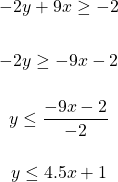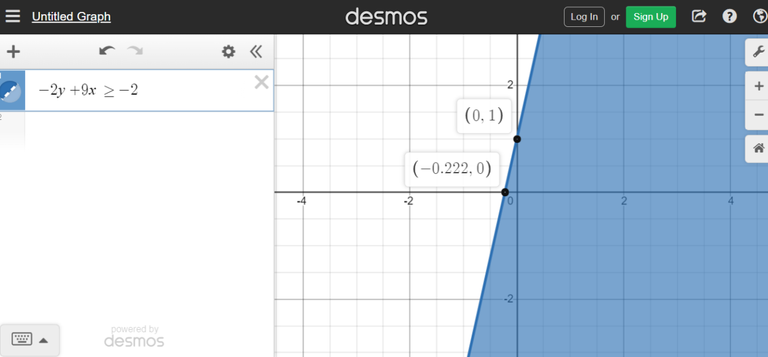Example Four - Phone Plan Charges

Suppose you buy a phone outright at its full costs. The cost for this new phone is about \$2700 CAD. To supplement the cost of this phone, the phone plan is a \$80 CAD phone plan per month. Keeping overage fees in mind, what would be total costs paid over time look like with a graph? The shaded region would represents total costs paid if overage fees occurred.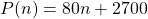where `n` is the number of months paid and `P(x)` is the total amount paid to the phone company at month `n`.

As we may incur extra fees, we have this inequality: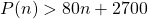The Desmos screenshot of this does not look great. Having a large slope of 80 makes the line steep to the point that it is almost vertical. The shaded region is above the dashed line is coloured blue.